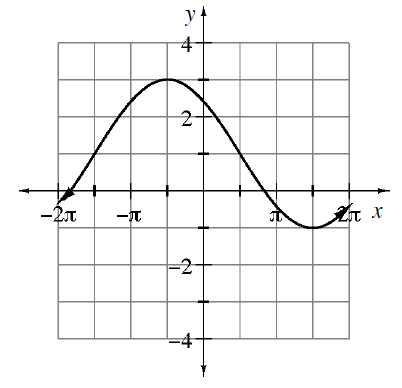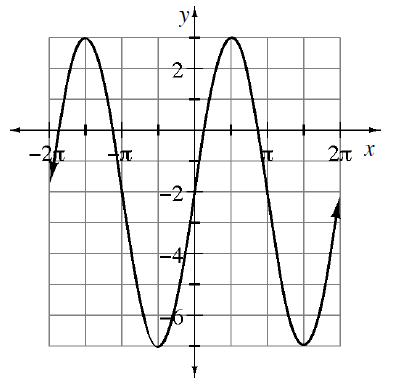### Home > INT3 > Chapter 12 > Lesson 12.1.4 > Problem12-67

12-67.

Write a possible equation for the graphs shown below. Then state the amplitude and period of the function. Describe how the graph relates to the parent function.

Look at the graph to determine the amplitude, period, phase shift, and vertical shift.

1. parent: $y=\sin(x)$Use the information in $y = a\ \text{sin}\ b\left(x + c\right) + d$

$y=-2 \text{ sin}\left (\frac{1}{2}\left (x-\frac{\pi}{2}\right )\right )+1$

1. parent: $y=\cos(x)$